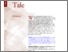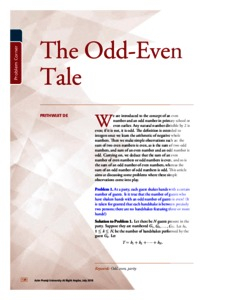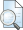# The odd-even tale

De, Prithwijit (2018) The odd-even tale. At Right Angles, 7 (2). pp. 126-129. ISSN 2582-1873Preview
Text - Published Version

## Abstract

We are introduced to the concept of an even number and an odd number in primary school or even earlier. Any natural number divisible by 2 is even; if it is not, it is odd. The definition is extended to integers once we learn the arithmetic of negative whole numbers. Then we make simple observations such as: the sum of two even numbers is even, as is the sum of two odd numbers, and sum of an even number and an odd number is odd. Carrying on, we deduce that the sum of an even number of even numbers or odd numbers is even, and so is the sum of an odd number of even numbers, whereas the sum of an odd number of odd numbers is odd. This article aims at discussing some problems where these simple observations come into play.

Item Type: Articles in APF Magazines
Authors: De, Prithwijit
Document Language:
Language
English
Uncontrolled Keywords: Odd, even, parity
Subjects: Natural Sciences > Mathematics
Divisions: Azim Premji University > University Publications > At Right Angles
Full Text Status: Public
URI: http://publications.azimpremjiuniversity.edu.in/id/eprint/1606
Publisher URL: http://apfstatic.s3.ap-south-1.amazonaws.com/s3fs-...View Item